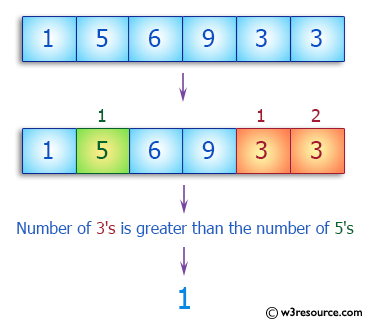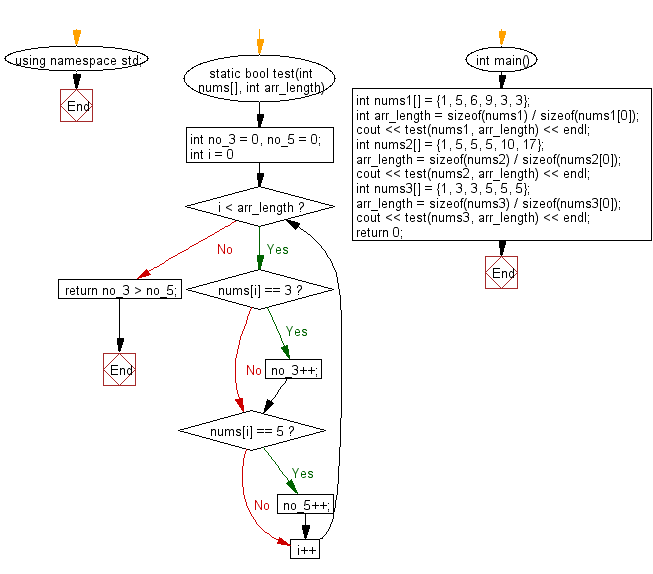﻿ C++ : Check if the number of 3s is greater than 5s# C++ Exercises: Check if the number of 3's is greater than the number of 5's

## C++ Basic Algorithm: Exercise-105 with Solution

Write a C++ program to check if the number of 3's is greater than the number of 5's.

Sample Solution:

C++ Code :

``````#include <iostream>
using namespace std;

static bool test(int nums[], int arr_length)
{
int no_3 = 0, no_5 = 0;

for (int i = 0; i < arr_length; i++)
{
if (nums[i] == 3) no_3++;
if (nums[i] == 5) no_5++;
}

return no_3 > no_5;
}

int main()
{
int nums1[] = {1, 5, 6, 9, 3, 3};
int arr_length = sizeof(nums1) / sizeof(nums1);
cout << test(nums1, arr_length) << endl;
int nums2[] = {1, 5, 5, 5, 10, 17};
arr_length = sizeof(nums2) / sizeof(nums2);
cout << test(nums2, arr_length) << endl;
int nums3[] = {1, 3, 3, 5, 5, 5};
arr_length = sizeof(nums3) / sizeof(nums3);
cout << test(nums3, arr_length) << endl;
return 0;
}
``````

Sample Output:

```1
0
0
```

Pictorial Presentation:Flowchart:C++ Code Editor:

Contribute your code and comments through Disqus.

What is the difficulty level of this exercise?

﻿